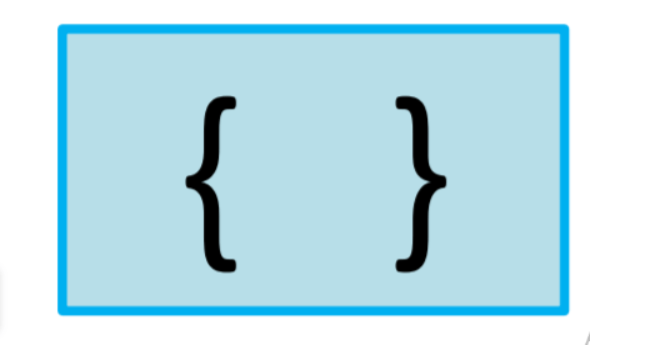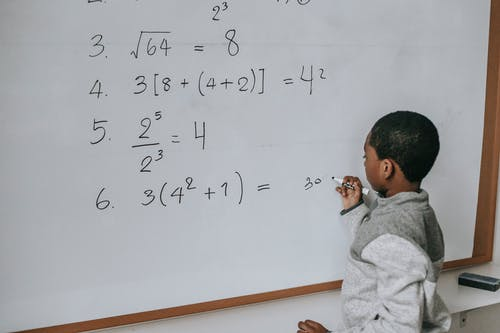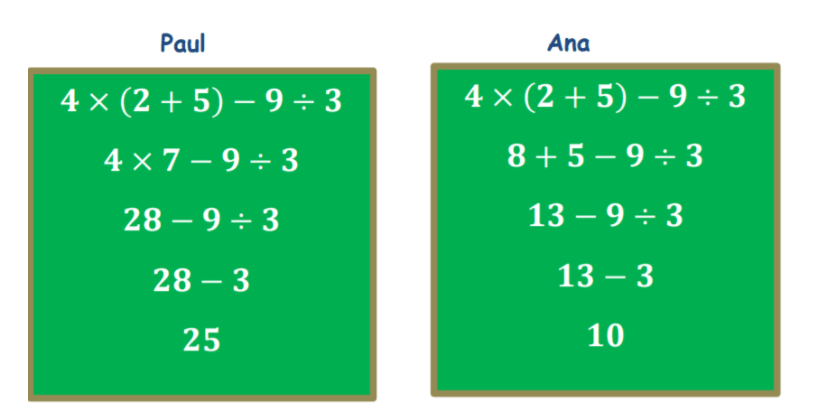# Teaching Order of Operations With Parentheses

 Listen to this Lesson:

When learning about the order of operations with parentheses in grade 5, students become fluent in evaluating numerical expressions with parentheses, as well in using the order of operations in solving problems that involve numerical expressions.

To make this topic more engaging for kids, math teachers can incorporate special teaching tips and fun games in class. We bring you a few such tips and games in this article. Use them in your teaching and get students excited about learning the order of operations with parentheses!

## Tips to Teach the Order of Operations With Parentheses### Review the Order of Operations

Start by reminding students what the word order and the word operations mean. Explain that the word ‘arrangement’ is a synonym for the word ‘order’. Then, point out that when we say ‘operations’ in math, we are referring to addition, subtraction, division, and multiplication.

Therefore, the ‘order of operations’ in math means the correct arrangement of the operations involved in a numerical expression. The order of operations is simply a set of math rules that tell us which operation (ex: multiplication, addition, etc.) to do first.

Students are familiar with the order of operations without parentheses, where we follow the MDAS rule. This rule tells us that first, we do multiplication or division, whichever comes first, and then addition or subtraction, whichever comes first, always from left to right.

In expressions where we don’t have any grouping symbols (ex: parenthesis), we can use the MDAS rule. Yet there are expressions that contain grouping symbols. How do we evaluate such expressions? Will we still use the MDAS rule? Ask students to think about this.

### Grouping Symbols

Point out that to be able to write an accurate numerical expression, we need to be able to first analyze the verbal statement accurately. Add that there are cases where we group together some numbers and operations with symbols.

Students already know of parenthesis as one such grouping symbol, but there are other symbols in addition to it. Ask students if they can think of any such other symbols, and then enumerate all such grouping symbols:

• parentheses• brackets• bracesPoint out that when there are expressions with certain symbols, there is an order that we have to observe. This means that we have to do the operations inside certain symbols before operations inside other symbols. You can present this order of symbols in the following way:

• We initially perform the operation in parentheses
• Then, we perform the operation in brackets
• Finally, we perform the operation in braces### Evaluating Numerical Expressions with Parentheses

Remind students that when we say that we want to evaluate an expression, we are basically talking about calculating its value. Point out that we will look into evaluating numerical expressions where we have grouping symbols.

Then, provide a worked example of how we evaluate these types of expressions. You can start with a simpler example, where we only have one grouping symbol, such as parentheses. For instance, write this expression on the whiteboard:

4 × (2 + 5) – 9 ÷ 3 = ?

Add that two classmates, one called Ana, and the other one Paul, both evaluated this numerical expression, but in two different ways. However, one answer is inaccurate. Present the two different solutions and ask students to reflect which student got it right:Explain that in order to correctly evaluate a numerical expression with grouping symbols, we have to follow some steps. Point out that we use the PEMDAS rule throughout these steps when we have expressions with brackets, braces, and parentheses.

#### What Is the PEMDAS rule?

Explain to students that the PEMDAS rule stands for parentheses – exponents – multiplication – division – addition – subtraction. There are a few steps that we need to observe when applying the PEMDAS rule. You can enumerate these steps on the whiteboard:

• Step 1:

First, we deal with the numbers and operations in parentheses, then those in brackets, leaving those in braces for the end.

• Step 2:

In cases where we have exponents, we evaluate the exponents.

• Step 3:

Once we’ve dealt with the calculations in parentheses, brackets and braces, and we got rid of the grouping symbols, we can proceed with the remaining operations. Point out that we always do this from left to right.

• Step 4:

We carry out multiplication or division. Add that it doesn’t matter which one we do first, and that we do the one that comes first when we are moving from left to right.

• Step 5:

We carry out addition or subtraction. Again, add that it doesn’t matter which one we do first, and that we do the one that comes first when we are moving from left to right.

Now go back to the solutions of Ana and Paul and analyze them. In Paul’s case, he first did the calculation inside the parentheses, by adding 2 and 5. He thus got: 4 × 7 – 9 ÷ 3 = ? and proceeded with multiplication and division, to get 28 – 3.

Finally, he carried out the subtraction, by subtracting 3 from 28 and obtained 25 as his solution to 4 × (2 + 5) – 9 ÷ 3. Highlight that Paul followed all the steps associated with the PEMDAS rule and his answer is correct.

Then, analyze Ana’s answer. She started off by multiplying 2 and 4 from the expression 4 × (2 + 5) – 9 ÷ 3 = ? and got 8 + 5 – 9 ÷ 3 = ? So her initial step was a mistake because she should have carried the operation inside the parentheses first. Her answer is thus incorrect.

#### Mixed Grouping Symbols Example:

Now that students have an understanding of how to evaluate simpler numerical expressions where we have one grouping symbol, such as parentheses, you can move on to providing an example of evaluating an expression with several grouping symbols, such as:

(10 + 2) ÷ 4 × [(153) – 4] = ?

Point out that first, we’ll deal with the numbers and operations inside the grouping symbols, starting with the ones that are in parentheses and then with the expression in brackets. This will result in the following:

12 ÷ 4 × [(15 ÷ 3) – 4] = ?

12 ÷ 4 × [5 – 4] = ?

12 ÷ 4 × 1 = ?

Since we’re done with all the calculations inside the grouping symbols, we’ll continue with the calculations from left to right. We’re left with division and multiplication and we can do the first one that comes from left to right, which is division in this case:

3 × 1 = 3

If you have the technical possibilities in your classroom, you can also enrich your lesson on teaching the order of operations with parentheses with multimedia material, such as videos on this topic.

For instance, you can use this video as an introduction to your lesson, and then continue with this video to provide a practical example on how to evaluate numerical expressions with parentheses using the order of operations.

## Activities to Practice the Order of Operations With Parentheses

### Evaluate Expressions With Parentheses Game

This is an online game where students will have the opportunity to hone their skills in evaluating numerical expressions with parentheses. To implement this game in your classroom, make sure there are enough technical devices for your class.

Students play the game individually, which makes it suitable for parents who are homeschooling their children as well. They are presented with different exercises where they have to use the PEMDAS rule to evaluate the expressions correctly.

Provide a few minutes for this, then open space for discussion and reflection. What does PEMDAS stand for? How did students apply the PEMDAS rule? Were any of the exercises particularly challenging?

### Order of Operations Game

In this online game, students will be able to practice evaluating numerical expressions that contain grouping symbols, as well as exponents. To play the game in your classroom, the only thing you’ll need are technical devices.

Explain to students that this is also an individual game, where they have to evaluate expressions by dealing with the given operations in each expression in the correct order. Students can also use a hint or watch a video if they get stuck.

In the end, allow time for students to share the thought process of how they solved each exercise. At what point did they find the value of numbers with exponents? How did they use the PEMDAS rule in each given expression?

## Before You Leave…

We hope you’ve enjoyed our methods and activities on teaching the order of operations with parentheses! Make sure to check out our worksheets and resources on how to teach this topic to 5th graders. These are all pdf files, so you’ll be able to print them out easily:

To get the Editable versions of these files, join us inside the Math Teacher Coach Community:

These activities are from:

Unit 1 – Numerical Expressions